### Shortest Path

A shortest path between two nodesandin a graph is a path that starts atand ends atwith the lowest total link weight. The starting node is referred to as the source node, and the ending node is referred to as the sink node.

In PROC OPTNET, shortest paths can be calculated by invoking the SHORTPATH statement. The options for this statement are described in the section SHORTPATH Statement.

By default, PROC OPTNET finds shortest paths for all pairs. That is, it finds a shortest path for each possible combination of source and sink nodes. Alternatively, you can use the SOURCE= option to fix a particular source node and find shortest paths from the fixed source node to all possible sink nodes. Conversely, by using the SINK= option, you can fix a sink node and find shortest paths from all possible source nodes to the fixed sink node. Using both options together, you can request one particular shortest path for a specific source-sink pair. In addition, you can use the DATA_NODES_SUB= option to define a list of source-sink pairs to process, as described in the section Node Subset Input Data. The following sections show examples of these options.

The algorithm used in PROC OPTNET for finding shortest paths is a variant of Dijkstra’s algorithm (Ahuja, Magnanti, and Orlin 1993). For unweighted graphs, PROC OPTNET uses a variant of breadth-first search. Dijkstra’s algorithm on weighted graphs runs in time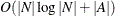for each source node. Breadth-first search runs in time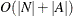for each source node.

For weighted graphs, the algorithm uses the weight variable that is defined in the links data set to evaluate a path’s total weight (or cost). You can also use the WEIGHT2= option in the SHORTPATH statement to define an auxiliary weight. The auxiliary weight is not used in the algorithm to evaluate a path’s total weight. It is simply calculated for the sake of reporting the total auxiliary weight for each shortest path.

#### Output Data Sets

The shortest path algorithm produces up to two output data sets. The output data set that is specified in the OUT_PATHS= option contains the links of a shortest path for each source-sink pair combination. The output data set that is specified in the OUT_WEIGHTS= option contains the total weight for the shortest path for each source-sink pair combination.

##### OUT_PATHS= Data Set

This data set contains the links present in the shortest path for each of the source-sink pairs. For large graphs and a large requested number of source-sink pairs, this output data set can be extremely large. In this case, the generation of the output can sometimes take longer than the computation of the shortest paths. For example, using the U.S. road network data for the state of New York, the data contain a directed graph with 264,346 nodes. Finding the shortest path for all pairs from only one source node results in 140,969,120 observations, which is a data set of size 11 GB. Finding shortest paths for all pairs from all nodes would produce an enormous output data set.

The OUT_PATHS= data set contains the following columns:

• source: the source node label of this shortest path

• sink: the sink node label of this shortest path

• order: for this source-sink pair, the order of this link in a shortest path

• from: the from node label of this link in a shortest path

• to: the to node label of this link in a shortest path

• weight: the weight of this link in a shortest path

• weight2: the auxiliary weight of this link

##### OUT_WEIGHTS= Data Set

This data set contains the total weight (and total auxiliary weight) for the shortest path for each of the source-sink pair.

The data set contains the following columns:

• source: the source node label of this shortest path

• sink: the sink node label of this shortest path

• path_weight: the total weight of the shortest path for this source-sink pair

• path_weight2: the total auxiliary weight of the shortest path for this source-sink pair

#### Shortest Paths for All Pairs

This example illustrates the use of the shortest path algorithm for all source-sink pairs on the simple undirected graph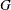shown in Figure 2.46.

Figure 2.46: A Simple Undirected Graph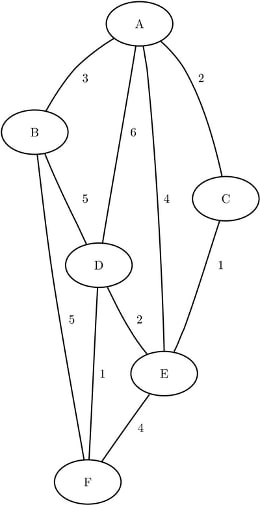The undirected graphcan be represented by the links data set LinkSetIn as follows:

data LinkSetIn;
input from $to$ weight @@;
datalines;
A B 3  A C 2  A D 6  A E 4  B D 5
B F 5  C E 1  D E 2  D F 1  E F 4
;


The following statements calculate shortest paths for all source-sink pairs:

proc optnet
shortpath
out_weights = ShortPathW
out_paths   = ShortPathP;
run;


The data set ShortPathP contains the shortest paths and is shown in Figure 2.47.

Figure 2.47: All-Pairs Shortest Paths

 ShortPathP

source sink order from to weight
A B 1 A B 3
A C 1 A C 2
A D 1 A C 2
A D 2 C E 1
A D 3 E D 2
A E 1 A C 2
A E 2 C E 1
A F 1 A C 2
A F 2 C E 1
A F 3 E D 2
A F 4 D F 1
B A 1 B A 3
B C 1 B A 3
B C 2 A C 2
B D 1 B D 5
B E 1 B A 3
B E 2 A C 2
B E 3 C E 1
B F 1 B F 5
C A 1 C A 2
C B 1 C A 2
C B 2 A B 3
C D 1 C E 1
C D 2 E D 2
C E 1 C E 1
C F 1 C E 1
C F 2 E D 2
C F 3 D F 1
D A 1 D E 2
D A 2 E C 1
D A 3 C A 2
D B 1 D B 5
D C 1 D E 2
D C 2 E C 1
D E 1 D E 2
D F 1 D F 1
E A 1 E C 1
E A 2 C A 2
E B 1 E C 1
E B 2 C A 2
E B 3 A B 3
E C 1 E C 1
E D 1 E D 2
E F 1 E D 2
E F 2 D F 1
F A 1 F D 1
F A 2 D E 2
F A 3 E C 1
F A 4 C A 2
F B 1 F B 5
F C 1 F D 1
F C 2 D E 2
F C 3 E C 1
F D 1 F D 1
F E 1 F D 1
F E 2 D E 2

The data set ShortPathW contains the path weight for the shortest paths of each source-sink pair and is shown in Figure 2.48.

Figure 2.48: All-Pairs Shortest Paths Summary

 ShortPathW

source sink path_weight
A B 3
A C 2
A D 5
A E 3
A F 6
B A 3
B C 5
B D 5
B E 6
B F 5
C A 2
C B 5
C D 3
C E 1
C F 4
D A 5
D B 5
D C 3
D E 2
D F 1
E A 3
E B 6
E C 1
E D 2
E F 3
F A 6
F B 5
F C 4
F D 1
F E 3

When you are interested only in the source-sink pair with the longest shortest path, you can use the PATHS= option. This option affects only the output processing; it does not affect the computation. All of the designated source-sink shortest paths are calculated, but only the longest ones are written to the output data set.

The following statements display only the longest shortest paths:

proc optnet
shortpath
paths     = longest
out_paths = ShortPathLong;
run;


The data set ShortPathLong now contains the longest shortest paths and is shown in Figure 2.49.

Figure 2.49: Longest Shortest Path

 ShortPathLong

source sink order from to weight
A F 1 A C 2
A F 2 C E 1
A F 3 E D 2
A F 4 D F 1
B E 1 B A 3
B E 2 A C 2
B E 3 C E 1
E B 1 E C 1
E B 2 C A 2
E B 3 A B 3
F A 1 F D 1
F A 2 D E 2
F A 3 E C 1
F A 4 C A 2

#### Shortest Paths for a Subset of Source-Sink Pairs

This section illustrates the use of a node subset data set, the DATA_NODES_SUB= option, and the shortest path algorithm for calculating shortest paths between a subset of source-sink pairs. The data set variables source and sink are used as indicators to specify which pairs to process. The marked source nodes define a set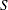, and the marked sink nodes define a set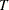. PROC OPTNET then calculates all the source-sink pairs in the cross product of these two sets.

For example, the following DATA step tells PROC OPTNET to calculate the pairs in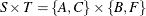:

data NodeSetInSub;
input node $source sink; datalines; A 1 0 C 1 0 B 0 1 F 0 1 ;  The following statements calculate a shortest path for the four combinations of source-sink pairs: proc optnet data_nodes_sub = NodeSetInSub data_links = LinkSetIn; shortpath out_paths = ShortPath; run;  The data set ShortPath contains the shortest paths and is shown in Figure 2.50. Figure 2.50: Shortest Paths for a Subset of Source-Sink Pairs  ShortPath source sink order from to weight A B 1 A B 3 A F 1 A C 2 A F 2 C E 1 A F 3 E D 2 A F 4 D F 1 C B 1 C A 2 C B 2 A B 3 C F 1 C E 1 C F 2 E D 2 C F 3 D F 1 #### Shortest Paths for a Subset of Source or Sink Pairs This section illustrates the use of the shortest path algorithm for calculating shortest paths between a subset of source (or sink) nodes and all other sink (or source) nodes. In this case, you designate the subset of source (or sink) nodes in the node subset data set by specifying source (or sink). By specifying only one of the variables, you indicate that you want PROC OPTNET to calculate all pairs from a subset of source nodes (or all pairs to a subset of sink nodes). For example, the following DATA step designates nodes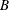and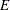as source nodes: data NodeSetInSub; input node$ source;
datalines;
B 1
E 1
;


You can use the same PROC OPTNET call as is used in the section Shortest Paths for a Subset of Source-Sink Pairs to calculate all the shortest paths from nodesand. The data set ShortPath contains the shortest paths and is shown in Figure 2.51.

Figure 2.51: Shortest Paths for a Subset of Source Pairs

 ShortPath

source sink order from to weight
B A 1 B A 3
B C 1 B A 3
B C 2 A C 2
B D 1 B D 5
B E 1 B A 3
B E 2 A C 2
B E 3 C E 1
B F 1 B F 5
E A 1 E C 1
E A 2 C A 2
E B 1 E C 1
E B 2 C A 2
E B 3 A B 3
E C 1 E C 1
E D 1 E D 2
E F 1 E D 2
E F 2 D F 1

Conversely, the following DATA step designates nodesandas sink nodes:

data NodeSetInSub;
input node $sink; datalines; B 1 E 1 ;  You can use the same PROC OPTNET call again to calculate all the shortest paths to nodesand. The data set ShortPath contains the shortest paths and is shown in Figure 2.52. Figure 2.52: Shortest Paths for a Subset of Sink Pairs  ShortPath source sink order from to weight A B 1 A B 3 A E 1 A C 2 A E 2 C E 1 B E 1 B A 3 B E 2 A C 2 B E 3 C E 1 C B 1 C A 2 C B 2 A B 3 C E 1 C E 1 D B 1 D B 5 D E 1 D E 2 E B 1 E C 1 E B 2 C A 2 E B 3 A B 3 F B 1 F B 5 F E 1 F D 1 F E 2 D E 2 #### Shortest Paths for One Source-Sink Pair This section illustrates the use of the shortest path algorithm for calculating shortest paths between one source-sink pair by using the SOURCE= and SINK= options. The following statements calculate a shortest path between node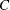and node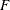: proc optnet data_links = LinkSetIn; shortpath source = C sink = F out_paths = ShortPath; run;  The data set ShortPath contains this shortest path and is shown in Figure 2.53. Figure 2.53: Shortest Paths for One Source-Sink Pair  ShortPath source sink order from to weight C F 1 C E 1 C F 2 E D 2 C F 3 D F 1 The shortest path is shown graphically in Figure 2.54. Figure 2.54: Shortest Path between Nodesand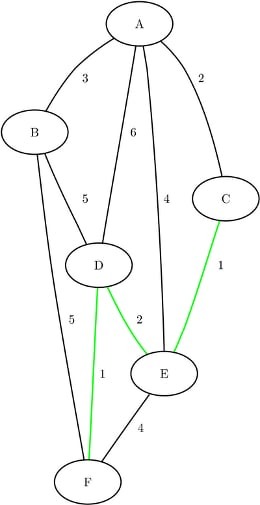#### Shortest Paths with Auxiliary Weight Calculation This section illustrates the use of the shortest path algorithm with auxiliary weights for calculating shortest paths between all source-sink pairs. Consider a links data set where the auxiliary weight is a counter for each link: data LinkSetIn; input from$ to \$ weight count @@;
datalines;
A B 3 1  A C 2 1  A D 6 1  A E 4 1  B D 5 1
B F 5 1  C E 1 1  D E 2 1  D F 1 1  E F 4 1
;


The following statements calculate shortest paths for all source-sink pairs:

proc optnet
shortpath
weight2    = count
out_weights = ShortPathW;
run;


The data set ShortPathW contains the total path weight for shortest paths in each source-sink pair and is shown in Figure 2.55. Since the variable count in LinkSetIn is 1 for all links, the value in the output data set variable path_weights2 gives the number of links in each shortest path.

Figure 2.55: Shortest Paths Including Auxiliary Weights in Calculation

 ShortPathW

source sink path_weight path_weight2
A B 3 1
A C 2 1
A D 5 3
A E 3 2
A F 6 4
B A 3 1
B C 5 2
B D 5 1
B E 6 3
B F 5 1
C A 2 1
C B 5 2
C D 3 2
C E 1 1
C F 4 3
D A 5 3
D B 5 1
D C 3 2
D E 2 1
D F 1 1
E A 3 2
E B 6 3
E C 1 1
E D 2 1
E F 3 2
F A 6 4
F B 5 1
F C 4 3
F D 1 1
F E 3 2

The section Road Network Shortest Path shows an example of using the shortest path algorithm for minimizing travel to and from work based on traffic conditions.